Courses

# Worksheet Question - Lines and Angles Class 7 Notes | EduRev

## Mathematics (Maths) Class 7

Created by: Praveen Kumar

## Class 7 : Worksheet Question - Lines and Angles Class 7 Notes | EduRev

The document Worksheet Question - Lines and Angles Class 7 Notes | EduRev is a part of the Class 7 Course Mathematics (Maths) Class 7.
All you need of Class 7 at this link: Class 7

Ques 1: (a) Complementary angle of 65° is .......................
(b) Supplementary angle of 45° is .........................
(c) If line AB||CD and LM is transversal, sum of two interior angles on the same side of transversal is equal to ......................
(d) An angle is formed by the intersection of ...........................
Ans: (a) 25°
(b) 135°
(c) 180°
(d) Two lines or two surfaces

Ques 2: In the figure, identify the pairs of corresponding angles.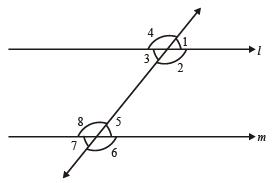Ans: ∠1 & ∠ 5, ∠2 &  ∠6, ∠4 & ∠8, ∠3 &  ∠7

Ques 3: An angle is greater than 45°. Its complement will be:
(a) Less than 45°
(b) Equal to 45°
(c) Greater than 45°
(d) None of these
Ans: (a)

Ques 4: State true of false:
(a) If measure fo an angle is 90° then its supplement angle will be greater than 90°.
(b) Two obtuse angles form a linear pair.
(c) Two acute angles form a linear pair.
(d) If two adjacent angles are complementary they form a right angle.
Ans: (a) False
(b) False
(c) False
(d) True

Ques 5: In the figure, the arms of two angles are parallel.
If PQR = 70°, then find
(i) LGR
(ii) LMN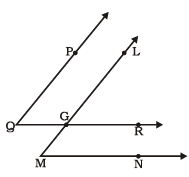Ans: (i) ∠LGR = 70°
(ii) ∠LMN = 70°

Ques 6: Indicate two pairs of angles which are:
(i) Vertically opposite angles
(ii) Linear pairs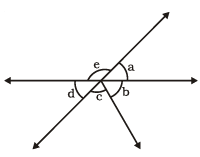Ans. (i) ∠a & ∠d
(ii) ∠a & ∠e, ∠e & ∠d

Ques 7: In the following figure, is x is adjacent to y?
Give reason.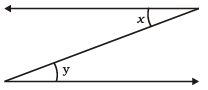Ans: No. because vertex is not common

Ques 8: Find the angle:
(i) Which is equal to its complement.
(ii) Which is equal to its supplement.
Ans. (i) 45°
(ii) 90°

Ques 9: In the given figure, decide a is parallel to b:
(i)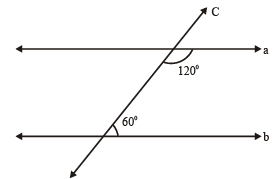(ii)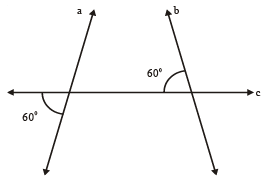(iii)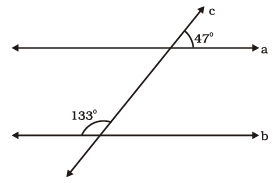Ans. (i) Yes
(ii) No
(iii) Yes

Ques 11: State the property that is used in each of the following statements:
(i) If l || m, then 4 =
(ii) If 1 = 7, then l || m
(iii) If 3 + 8 = 180°, then l||m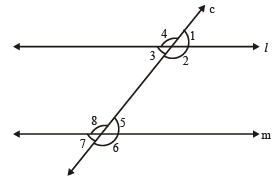Ans. (i) Correspondign angles
(ii) Corresponding & vertically opposite angles
(iii) Linear Pair

Ques 11: Find the value of y in each of the following figure if a || b:
(i)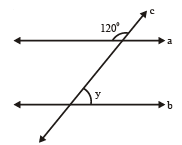(ii)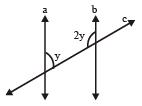(iii)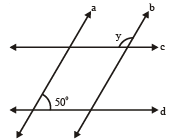Ans: (i) y = 60°
(ii) y = 60°
(iii) y = 130°

Ques 12: In the following figure name the following pairs of angles:
(i) Obtuse vertically opposite angles.
(iii) Euqal supplementary angles.
(iv) Unequal supplementary angles.
(v) Adjacement angles that do not form a linear pair.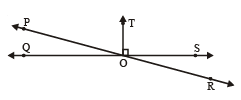Ans. (i) ∠POS & ∠QOR
(ii) ∠QOP & ∠POT
(iii) ∠QOT & ∠TOS
(iv) ∠POQ & ∠QOR, ∠POQ & ∠POS
(v) ∠TOS & ∠SOR, ∠POQ & ∠POT, ∠POT & ∠TOS

,

,

,

,

,

,

,

,

,

,

,

,

,

,

,

,

,

,

,

,

,

;